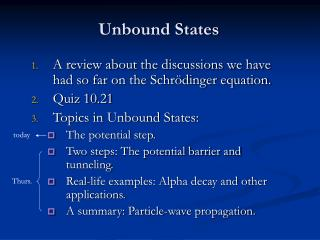Download PresentationUnbound States

# Unbound States - PowerPoint PPT Presentation

Unbound States. today. A review about the discussions we have had so far on the Schrödinger equation. Quiz 10.21 Topics in Unbound States: The potential step. Two steps: The potential barrier and tunneling. Real-life examples: Alpha decay and other applications.I am the owner, or an agent authorized to act on behalf of the owner, of the copyrighted work described.
Download Presentation## Unbound States

An Image/Link below is provided (as is) to download presentation

Download Policy: Content on the Website is provided to you AS IS for your information and personal use and may not be sold / licensed / shared on other websites without getting consent from its author.While downloading, if for some reason you are not able to download a presentation, the publisher may have deleted the file from their server.

- - - - - - - - - - - - - - - - - - - - - - - - - - E N D - - - - - - - - - - - - - - - - - - - - - - - - - -
Presentation Transcript
1. Unbound States today A review about the discussions we have had so far on the Schrödinger equation. Quiz 10.21 Topics in Unbound States: The potential step. Two steps: The potential barrier and tunneling. Real-life examples: Alpha decay and other applications. A summary: Particle-wave propagation. Thurs.

2. Review: The Schrödinger equation The free particle Schrödinger Equation: The plane wave solution completely defined. The momentum of this particle: undefined. The location of this particle: From and We understand this equation as energy accounting And this leads to the equation that adds an external potential The Schrödinger Equation  Solve for with the knowledge of , for problems in QM.

3. Review: the time independent Schrödinger equation and the two conditions for the wave function When the wave function can be expressed as We have found and the time independent Schrödinger Equation:  The solution of this equation is the stationary states because The probability of finding a particle does not depend on time: Normalization: Two conditions The wave function be smooth  the continuity of the wave function and its first order derivative.

4. Review: Solving the Schrödinger equation. Case 1: The infinite potential well Equation and Solution: Energy and probability • Standing wave. • The QM ground-state. A bound state particle cannot be stationary, although its wave function is stationary. • Energy ratio at each level: n2. • With very large n, QM  CM.

5. The change  Solving the Schrödinger equation. Case 2: The finite potential well The change  Equations:

6. Penetration depth: Solutions: Energy quantization: Review: Solving the Schrödinger equation. Case 2: The finite potential well

7. Solve for wave function and energy level Review: Solving the Schrödinger equation. Case 3: The simple harmonic oscillator This model is a good approximation of particles oscillate about an equilibrium position, like the bond between two atoms in a di-atomic molecule.

8. Energy are equally spaced, characteristic of an oscillator Review Solving the Schrödinger equation. Case 3: The simple harmonic oscillator Wave function at each energy level Gaussian

9. Wave function: Wave function: Energy levels: Energy levels: Energy levels: Penetration depth: Compare Case 1, Case 2 and 3:

10. Re-write it in the form Right moving. Why? Right moving (along positive x-axis) wave of free particle: Left moving wave of free particle: New cases, unbound states: the potential step From potential well to a one-side well, or a step: Free particle with energy E. Standing waves do not form and energy is no quantized. We discussed about free particle wave function before. Which is:

11. The Schrödinger Equation: When When When Solution: The potential step: solve the equation Initial condition: free particles moving from left to right. Why no reflection here? Trans. Inc. Refl. Ans: no 2nd potential step on the right. Normalization and wave function smoothness Are we done? What do we learn here? Any other conditions to apply to the solutions?

12. The potential step: apply conditions Smoothness requires:   Express B and C in terms of A: Transmission probability: Reflection probability:

13. Transmission probability:  Reflection probability:  Reference: for normal incidence, light transmission probability: n air Reflection probability: The potential step: transmission and reflection Now using the definitions of k and k’:

14. The Schrödinger Equation: When When When Solution: Inc. Refl. The potential step: solve the equation Initial condition: free particles moving from left to right.

15.  Express B in terms of A: One can prove: Reflection probability: Penetration depth: The potential step: apply conditions Smoothness requires: Transmission probability:

16. Review questions • The plane wave solution of a free particle Schrödinger Equation is Can you normalize this wave function? • Try to solve for the wave function and discuss about transmission and reflection for this situation:

17. Preview for the next class (10/23) • Text to be read: • In chapter 6: • Section 6.2 • Section 6.3 • Section 6.4 • Questions: • How much do you know about radioactive decays of isotopes? Have you heard of alpha decay, beta decay and gamma sources? • Have you heard of the “tunneling effect” in the EE department (only for EE students)? • What is a wave phase velocity? What is a wave group velocity?

18. Homework 8, due by 10/28 • Problem 25 on page 187. • Problem 34 on page 188. • Problem 15 on page 224. • Problem 18 on page 224.